# Pandas DataFrame Plot bar graph

Pandas plot

Python Pandas Plot horizontal or vertical Bar graph by using DataFrame with options & save as image

Pandas.DataFrame.plot to get bar graphs using data

Let us create a DataFrame with name of the students and their marks.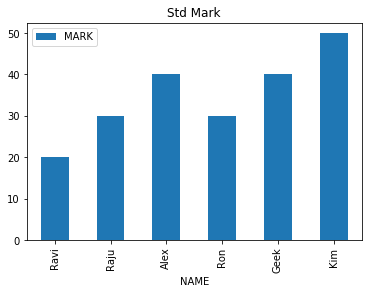``````import pandas as pd
my_dict={
'NAME':['Ravi','Raju','Alex',
'Ron','Geek','Kim'],
'MARK':[20,30,40,30,40,50]
}
df = pd.DataFrame(data=my_dict)
df.plot.bar(title="Std Mark",
x='NAME')``````

## Bar Graph with options

There are several options we can add to above bar graph.

## title :

title='Student Mark' String used as Title of the graph. We can also use one list to give titles to sub graphs. ( for this subplot must be true )

## figsize :

Size of the graph , it is a tuple saying width and height in inches, figsize=(6,3). Here width is 6 inches and height is 3 inches.

## X: Y:

What is to be used in X and Y axis. If you have multiple keys then you can specify what can be used. Note that Y axis must be numeric data to plot the graph.
Example : Here we have used y='MARK' to plot the graph against the name of students ( x='NAME' )
``df.plot.bar(title="Std Mark",x='NAME',y='MARK',figsize=(6,3));``

## use_index

True or False ( default is True ) , To show index ( use_index = True) or not ( use_index = False). The difference is mentioned here ( check X - axis ) .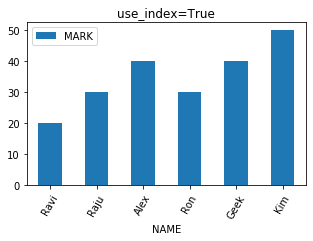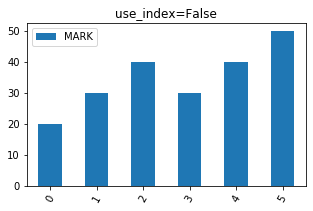## grid

We will show grid ( grid=True ) or not ( grid=False)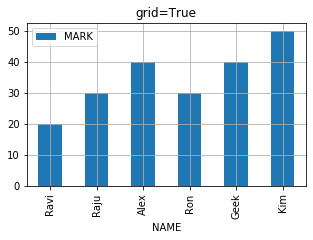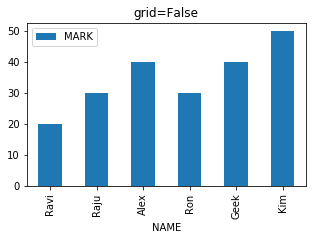## legend

True of False ( default is True ), to show legend ( legend=True ) or not ( legend=False)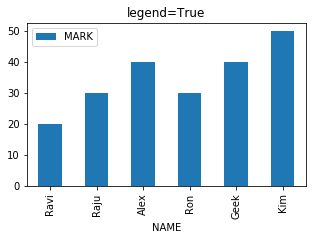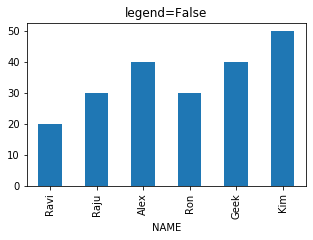## color

The colour of the bar we can define by using list. We have to give our inputs in R G B where each value varies from 0 to 1. Here is one sample.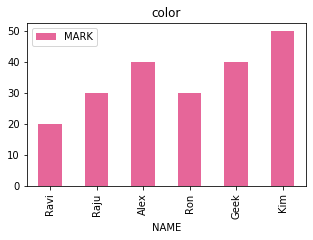code is here .
``````my_colors=[(0.9,0.4,0.6)]
df.plot.bar(title="color",x='NAME',
y='MARK',figsize=(5,3),
color=my_colors)``````

We can define more colours in combination of RGB and use them.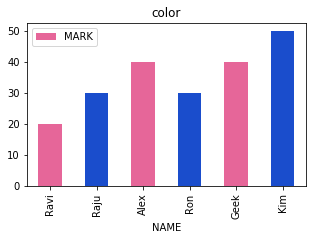``````my_colors=[(.9,.4,.6),(.1,.3,.8)]
df.plot.bar(title="color",x='NAME',
y='MARK',figsize=(5,3),
color=my_colors)``````

We can define different colours to each bar of the graph. We will prepare a list of different colours for each bar.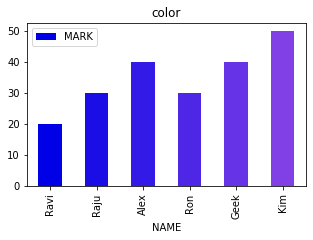``````my_colors = [(x/10.0, x/20.0, .9)
for x in range(len(df))]
df.plot.bar(title="color",x='NAME',
y='MARK',figsize=(5,3),
color=my_colors)``````

## logx logy loglog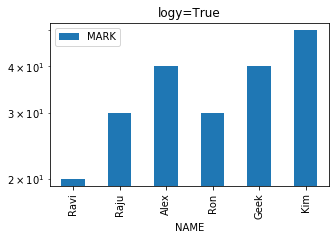We can specify log scaling or symlog scaling for x ( logx=True ) or y ( logy=True ) or for both x & y ( loglog=True)

``df.plot.line( title="logy=True",logy=True)``

## secondary_y

Whether to plot on secondary Y Axis ( secondary_y=True ) or not ( secondary_y=False )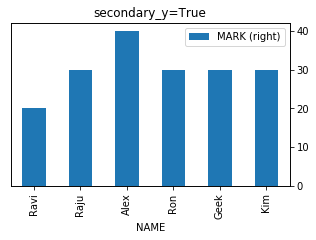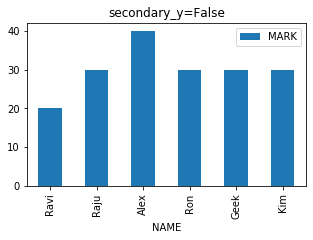## mark_right

Check the image above when secondary_y=True. There is a automatic marking in column lebels saying (right). We can manage this to show ( mark_right=True) or not ( mark_right=False)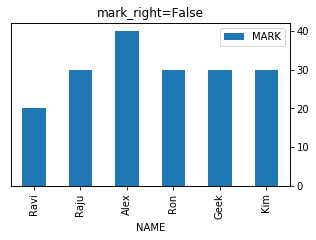``df.plot.bar( title="secondary_y=True",x='NAME',figsize=(5,3),secondary_y=True)``

## rot

Rotation of ticks ( check the names at x axis )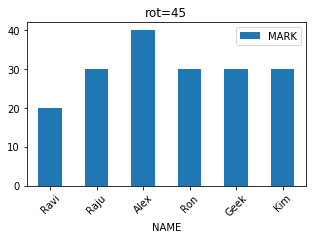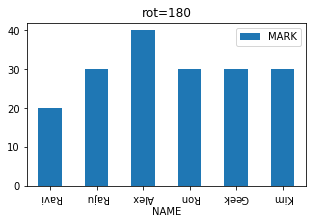``df.plot.bar( title="rot=180",x='NAME',figsize=(5,3),rot=180)``

## stacked

We can make bars stacked one over other ( stacked=True ) or not to draw side by side ( stacked=False)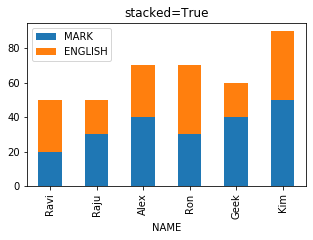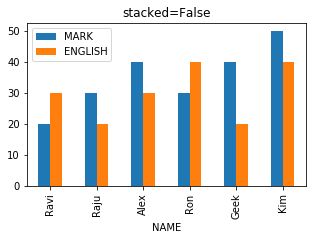``````import pandas as pd
my_dict={
'NAME':['Ravi','Raju','Alex',
'Ron','Geek','Kim'],
'MARK':[20,30,40,30,40,50],
'ENGLISH':[30,20,30,40,20,40]
}
df = pd.DataFrame(data=my_dict)
df.plot.bar(title="stacked=True",x='NAME',figsize=(5,3),stacked=True)``````

Subscribe to our YouTube Channel here

## Subscribe

* indicates required
Subscribe to plus2netplus2net.com